# Vector OperationsVector Operations: The vectors can be added, subtracted or multiplied with one another. Addition of vectors, difference of vectors, multiplication of a vector by a scalar, product of vectors, etc. are called vector operations.

********************

10 Math Problems officially announces the release of Quick Math Solver, an Android App on the Google Play Store for students around the world.

********************Study the following three cases:

Case I:Case II:Case III: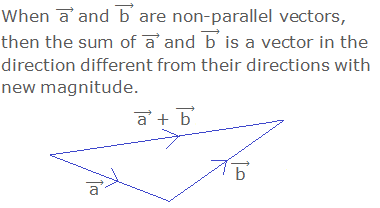## Triangle Law of Vector AdditionDefinition:

The triangle law of vector addition states that: “If the magnitude and direction of two vectors are represented by two sides of a triangle taken in order, then the magnitude and direction of their sum is given by the third side taken in reverse order.

## Parallelogram Law of Vector AdditionDefinition:

The parallelogram law of vector addition states that: “If two adjacent sides of a parallelogram through a point represents two vectors in magnitude and direction, then their sum is given by the diagonal of the parallelogram through the same point in magnitude and direction.

## Polygon Law of Vector AdditionDefinition:

The polygon law of vector addition states that: “If a number of vectors can be represented in magnitude and direction by the sides of polygon taken in order, their sum is given in magnitude and direction by the closing side of the polygon taken in reverse order.

## Addition of Two Vectors in Components Form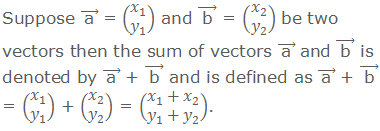### Geometrical Proof:## Subtraction of Vectors## Multiplication of a Vector by a Scalar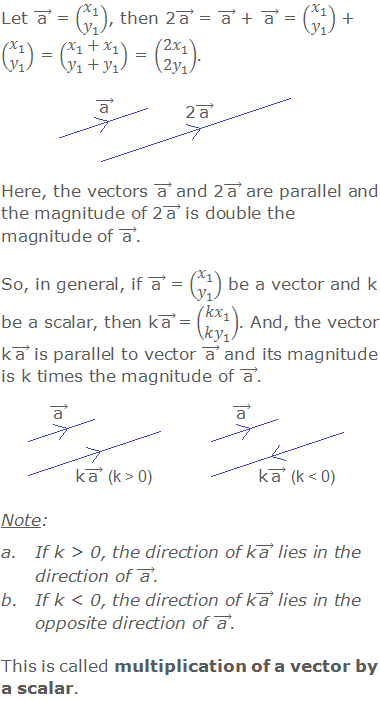## Unit Vectors i And j### Worked Out Examples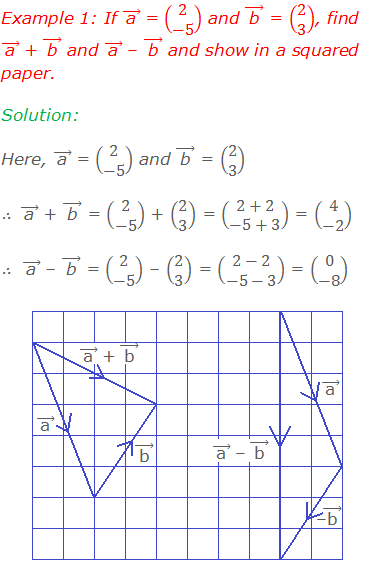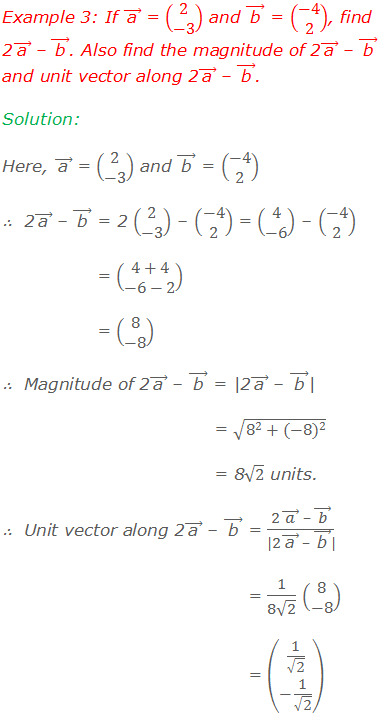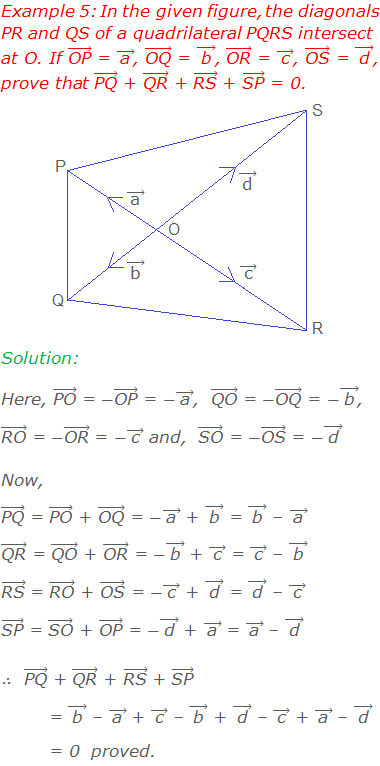#### Do you have any questions regarding the vector operations?

You can ask your questions or problems here, in the comment section below.